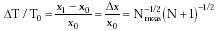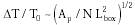Nanomedicine, Volume I: Basic Capabilities

Robert A. Freitas Jr., Nanomedicine, Volume I: Basic Capabilities, Landes Bioscience, Georgetown, TX, 1999

4.6.2 Piston-Based Temperature Sensors

Consider a coiled cylinder of cross-sectional area A filled with gas at pressure P, with a piston at one end whose movement is resisted by a constant-force spring (Fig. 4.7). Increasing gas temperature from the coldest temperature at which the sensor will operate (piston at maximum ingress), T0, to some warmer temperature T1 causes the piston to move from position x0 to position x1 while the spring holds pressure constant at P inside the cylinder during the measurement. If Dx is the smallest measurable piston displacement and DT = T1 - T0, then the poorest accuracy is{Eqn. 4.35}

because Dx has a thermal noise component as well,10 and the number of gas molecules N = nd Vsensor where nd ~ 1028 gas molecules/m3 at P = 1000 atm (Table 10.2). Sensitivity is maximized at the largest feasible x0; for DT / T0 = 10-6 (~300 microkelvins at 310 K) and Dx = Dxmin = 1 nm (Section 4.2.1), then x0 = 1000 microns, N = 109 gas molecules at 1000 atm (using the van der Waals equation of state) and 310 K taking Nmeas = 1000, giving Vsensor = 10-19 m3 and thus a cylinder cross-sectional area of A = Vsensor / x0 = (10 nm)2. There are ~1000 gas molecules per nanometer of cylinder length, each traveling with mean thermal velocity vt ~ 500 m/sec (Eqn. 3.3). Tightly coiled into a cubical volume, the folded sensor size is Lsensor ~ Vsensor1/3 ~ (464 nm)3 and measurement time tmeas = Nmeas Dtmin ~ 1 microsec. Sensor mass is ~10-16 kg.

The coiled tube design, though not strictly necessary, is nonetheless convenient because it allows a continuous sensor element to be arbitrarily distributed through the nanorobot volume, to be concentrated in multiple specific interior regions, or to be placed inside nanorobot components that will be required to flex such as metamorphic protuberances. If instead the working gas is placed in a cubical box of side Lbox with a sensor piston of area Ap, any change in temperature causes the piston to move a distance Dx = (Lbox3/Ap) (DT / T0) ~ (k T0 / ks)1/2, where ks = (Ap/Lbox2) (N kT0 / Lbox2), from Drexler;10 hence{Eqn. 4.36}

which argues for a small piston, a large box, and a high operating pressure to achieve maximum sensitivity. For Ap = 100 nm2, N = 109 molecules, and Lbox = 464 nm, DT / T ~ 10-6.

Last updated on 17 February 2003